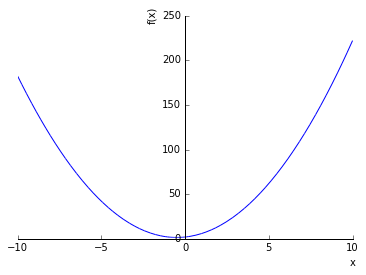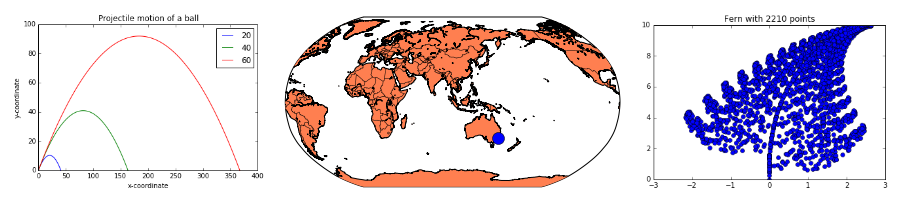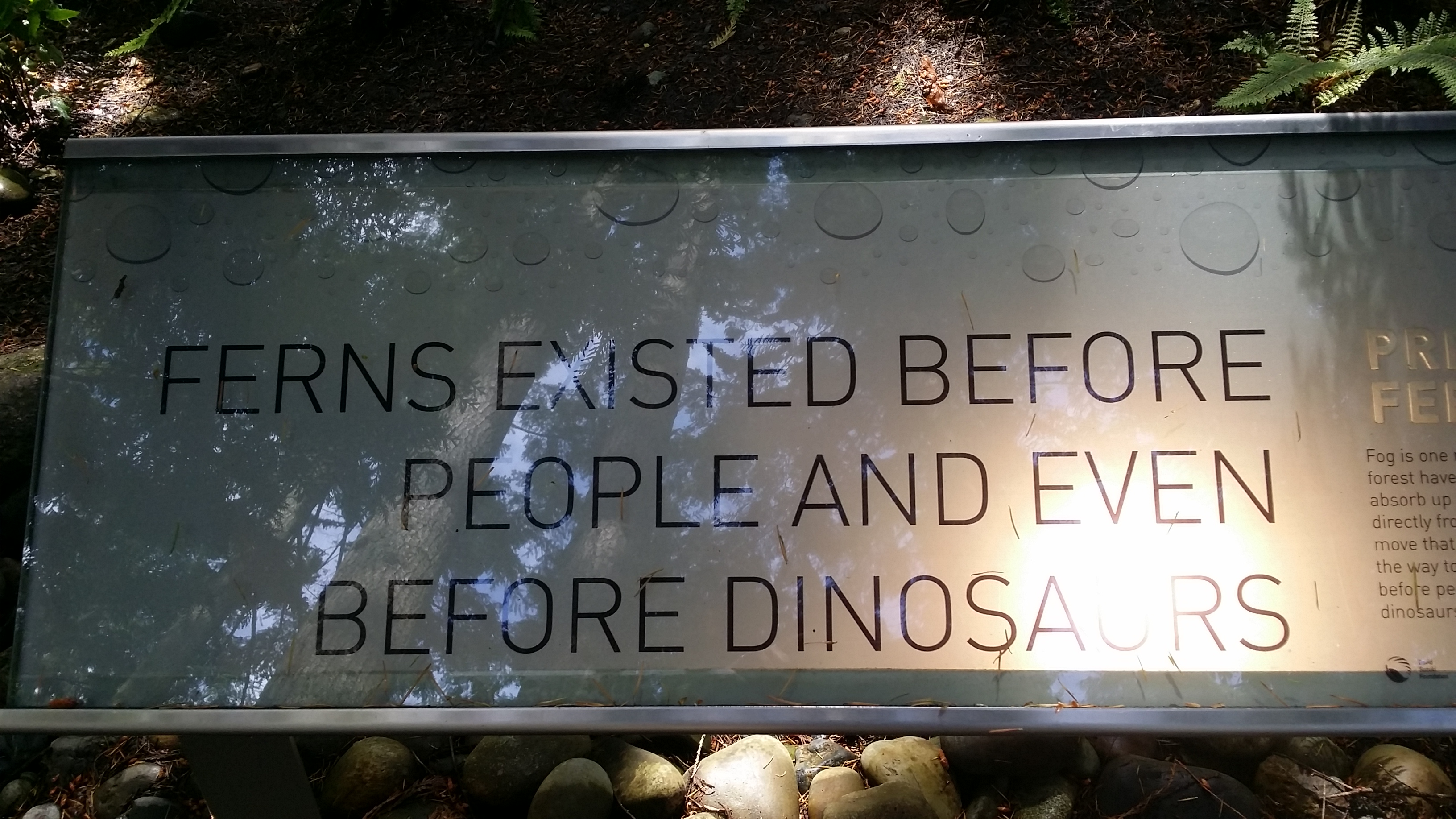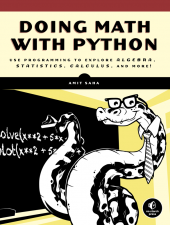# Doing Math with Python ¶

Amit Saha

May 29, PyCon US 2016 Education Summit

Portland, Oregon

• Software Engineer at Freelancer.com HQ in Sydney, Australia

• Author of "Doing Math with Python" (No Starch Press, 2015)

• Writes for Linux Voice, Linux Journal, and others.

#### Contact¶

Slides: https://doingmathwithpython.github.io/pycon-us-2016/

All presentation materials: https://github.com/doingmathwithpython

### This talk - a proposal, a hypothesis, a statement¶

What? Python can lead to a more enriching learning and teaching experience in the classroom

How? Next slides

### Tools (or, Giant shoulders we will stand on)¶Python 3, SymPy, matplotlib

*Individual logos are copyright of the respective projects. Source of the "giant shoulders" image.

### Python - a scientific calculator¶

Whose calculator looks like this?

>>> (131 + 21.5 + 100.2 + 88.7 + 99.5 + 100.5 + 200.5)/4
185.475


Python 3 is my favorite calculator (not Python 2 because 1/2 = 0)

Beyond basic operations:

• fabs(), abs(), sin(), cos(), gcd(), log() and more (See math)

• Descriptive statistics (See statistics)

### Python - a scientific calculator¶

• Develop your own functions: unit conversion, finding correlation, .., anything really

• Use PYTHONSTARTUP to extend the battery of readily available mathematical functions

\$ PYTHONSTARTUP=~/work/dmwp/pycon-us-2016/startup_math.py idle3 -s


### Unit conversion functions¶

>>> unit_conversion()
1. Kilometers to Miles
2. Miles to Kilometers
3. Kilograms to Pounds
4. Pounds to Kilograms
5. Celsius to Fahrenheit
6. Fahrenheit to Celsius
Which conversion would you like to do? 6
Enter temperature in fahrenheit: 98
Temperature in celsius: 36.66666666666667
>>>


### Finding linear correlation¶

>>>
>>> x = [1, 2, 3, 4]
>>> y = [2, 4, 6.1, 7.9]
>>> find_corr_x_y(x, y)
0.9995411791453812


### Python - a really fancy calculator¶

SymPy - a pure Python symbolic math library

from sympy import awesomeness - don't try that :)

In :
# Create graphs from algebraic expressions

from sympy import Symbol, plot
x = Symbol('x')
p = plot(2*x**2 + 2*x + 2)In :
# Solve equations

from sympy import solve, Symbol
x = Symbol('x')
solve(2*x + 1)

Out:
[-1/2]
In :
# Limits

from sympy import Symbol, Limit, sin
x = Symbol('x')
Limit(sin(x)/x, x, 0).doit()

Out:
1
In :
# Derivative

from sympy import Symbol, Derivative, sin, init_printing
x = Symbol('x')
init_printing()
Derivative(sin(x)**(2*x+1), x).doit()

Out:
$$\left(\frac{\left(2 x + 1\right) \cos{\left (x \right )}}{\sin{\left (x \right )}} + 2 \log{\left (\sin{\left (x \right )} \right )}\right) \sin^{2 x + 1}{\left (x \right )}$$
In :
# Indefinite integral

from sympy import Symbol, Integral, sqrt, sin, init_printing
x = Symbol('x')
init_printing()
Integral(sqrt(x)).doit()

Out:
$$\frac{2 x^{\frac{3}{2}}}{3}$$
In :
# Definite integral

from sympy import Symbol, Integral, sqrt
x = Symbol('x')
Integral(sqrt(x), (x, 0, 2)).doit()

Out:
$$\frac{4 \sqrt{2}}{3}$$

# Can we do more than write smart calculators?

### Python - Making other subjects more lively¶• matplotlib

• basemap

• Interactive Jupyter Notebooks

#### Bringing Science to life¶

Animation of a Projectile motion (Python Source)

In :
from IPython.display import YouTubeVideo

Out:

#### Exploring Fractals in Nature¶

Interactively drawing a Barnsley Fern (Notebook)#### The world is your graph paper¶

Showing places on a digital map (Notebook)

### Book: Doing Math With Python¶Overview

• All of what I have discussed so far

• In addition: Descriptive statistics, Sets and Probability, Random numbers

Upcoming/In-progress translations: Simplified Chinese, Japanese, French and Korean.

Saha does an excellent job providing a clear link between Python and upper-level math concepts, and demonstrates how Python can be transformed into a mathematical stage. This book deserves a spot on every geometry teacher’s bookshelf.

School Library Journal

Outstanding guide to using Python to do maths. Working back through my undergrad maths using Python.

Saha does an excellent job providing a clear link between Python and upper-level math concepts, and demonstrates how Python can be transformed into a mathematical stage.

This book is highly recommended for the high school or college student and anyone who is looking for a more natural way of programming math and scientific functions

As a teacher I highly recommend this book as a way to work with someone in learning both math and programming

### Great base for the future¶

Statistics and Graphing data -> Data Science

Differential Calculus -> Machine learning

### Application of differentiation¶

Use gradient descent to find a function's minimum value (Notebook)

### Predict the college admission score based on high school math score¶

Use gradient descent as the optimizer for single variable linear regression model (Notebook)

### Dialogue¶

#### Online¶

• Email: amitsaha.in@gmail.com

### PyCon Special!¶

Use PYCONMATH code to get 30% off "Doing Math with Python" from No Starch Press(Valid from May 26th - June 8th)

Book Signing - May 31st - 2.00 PM - No Starch Press booth

### Acknowledgements¶

PyCon US Education Summit team for inviting me

Thanks to PyCon US for reduced registration rates

Massive thanks to my employer, Freelancer.com for sponsoring my travel and stay